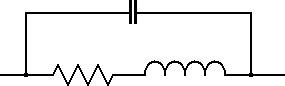# Inductor and Resistor in Series with Parallel Capacitor Calculator

This calculator finds the complex impedance (real and imaginary imaginary values) of an inductor and resistor in series with a parallel capacitor.Frequency: MHz kHz Hz Capacitance: uF nF pF Inductance: mH uH nH Resistance: MΩ kΩ Ω Z =

The complex impedance (Z) (real and imaginary) of an inductor and a resistor in series with a parallel capacitor at a particular frequency can be calculated using the following equations.Where:

f is the Frequency in Hz

C is the Capacitance in Farads

L is the Inductance in Henries

R is the Resistance in Ohms

This calculator uses JavaScript and will function in most modern browsers. For more information see About our calculators

This calculator is provided free by Chemandy Electronics in order to promote the FLEXI-BOX